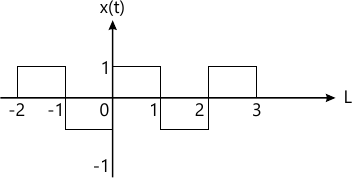MORE IN Signals & Systems
Total marks: --
Total time: --
INSTRUCTIONS
(1) Assume appropriate data and state your reasons
(2) Marks are given to the right of every question
(3) Draw neat diagrams wherever necessary

Answer any one question from Q1 and Q2
1 (a) Perform the following operations on the given signal x(t) which is defined as:

i) Sketch the signal x(t)
ii) Sketch z(t)= x(-t -1).
4 M
1 (b) Determine whether the following signals are periodic or not, if periodic find the fundamental period of the signal:
i) x(t) = cos (2t) + sin (2t) $x[n]= \cos \left ( \dfrac {8 \pi n}{15} \right )$
4 M
1 (c) Determine the step response of the following systems whose impulse response is:
h(t)=e-5t u(t)
4 M

2 (a) Compute the convolution integral by graphical method and sketch the output for
 x1(t)=1 0 ≤ t ≤ 2 0, otherwise x2(t)=e-2t u(t)
6 M
2 (b) Find even and odd component of
i) x(t) = u(t)
x(t) = sgn (t).
4 M
2 (c) Determine the whether following signal is periodic or not, if periodic find the the fundamental periodic of the signal.
x(t)=cos2 (2 πt).
2 M

Answer any one question from Q3 and Q4
3 (a) Find the trigonometric Fourier series for the periodic signal x(t). Sketch the amplitude and phase spectra.6 M
3 (b) A signal x(t) has Laplace transform $X(s)= \dfrac {s+1}{s^2 + 4s + 5 }$ Find the Laplace transform of the following signals:
i) y1(t)=t x(t)
ii) y2(t) = e-tx (t)
6 M

4 (a) Find the Fourier transform of $x(t) =rect \ \left ( \dfrac {t} {\tau} \right )$ and sketch the magnitude and phase spectrum.
6 M
4 (b) Find the transfer function of the following:
i) An ideal differentiator
ii) An ideal integrator
iii) An ideal delay of T second.
6 M

Answer any one question from Q5 and Q6
5 (a) Find the following for the give signal x(t):
i) Autocorrelation
ii) Energy from Autocorrelation
iii) Energy Spectral Density:
x(t)=Ae-at u(t).
6 M
5 (b) Determine the corss correlation between two sequences which are given below:
x1(n)={1 2 3 4}
x2(n)= {3 2 1 0}
4 M
5 (c) State and describe any three properties of Energy Spectral Density (ESD).
3 M

6 (a) Prove that autocorrelation and energy spectral density form Fourier transform pair of each other and verify the same for x(t) = e-2t u(t).
9 M
6 (b) State and explain any four properties of Power Spectral Density (PSD).
4 M

Answer any one question from Q7 and Q8
7 (a) Explain Gaussian probability model with respect to its density and distribution function.
4 M
7 (b) Two cards drawn from a 52 card deck successively without replacing the first:
i) Given the first one is heart, what is the probability that second is also a heart?
ii) What is the probability that both cards will be hearts?
4 M
7 (c) A coin is tossed three times. Write the sample space which gives all possible outcomes. A random variable X, which represents the number of heads obtained on any double toss. Draw the mapping of S on to real line. Also find the probabilities of X and plot the C.D.F.
5 M

8 (a) A random variable X is
 fx(X) = 5X2 ; 0 ≤ x ≤ 1 = 0    ; elsewhere

Find E[X], E[3X-2], E[X2].
6 M
8 (b) A student arrives late for a class 40% of the time. Class meets five times each week. Find:
i) Probability of students being late for at three classes in a given week.
ii) Probability of students will not be late at all during a given week.
4 M
8 (c) State the properties of Probability Density Function (PDF).
3 M

More question papers from Signals & Systems